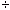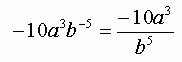From: Kristine I am having a hard time understanding factoring and multiplying polynomials. I have a couple of problems I just can't get, can you please help me? Simplify, do not use negatives in the answer:     (5a-1b-7)(-2a4b2) and:     (5x2y-7z)(-4xy-3z-4) Hi Kristine. There are two key ideas to learn here. The first is that when multiplying numbers with the same base, you add their exponents. For the first question, this means that you multiply the scalars together, multiply the a terms by adding their exponents, and do the same with the b terms: (5a-1b-7)(-2a4b2) = -10a-1 + 4b-7 + 2 = -10a3b-5 . The second key idea is derived from the first one: a negative exponent is the same as dividing by the positive value. When you are dividing two quantities with the same base, say c, you subtract their exponents: c6c2 = c4 . This means that to turn your negative exponents into positive ones, you make your answer a fraction with those term(s) in the denominator:Use the same principle to solve the second question, Kristine. Hope this helps,Stephen La Rocque.